# Ch6 z5e thermo

15 Jan 2011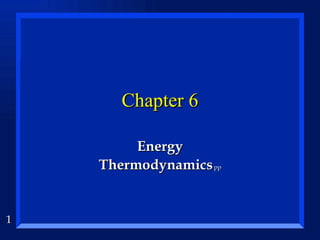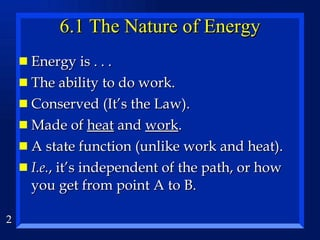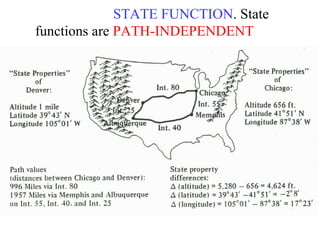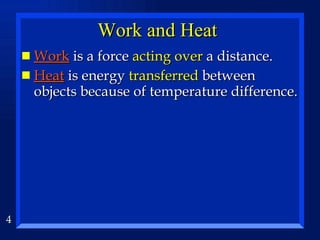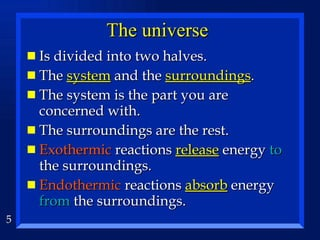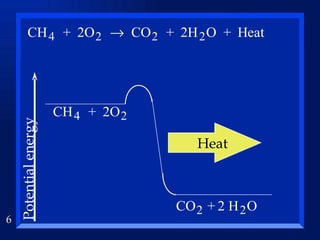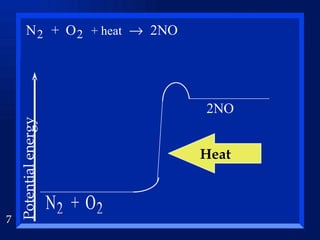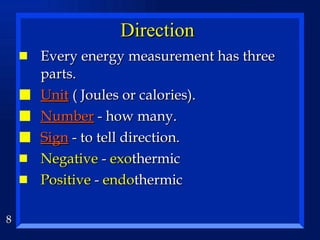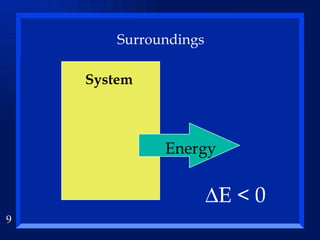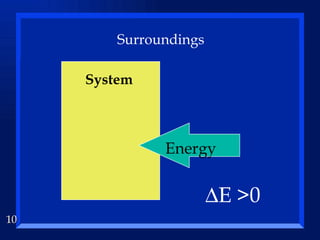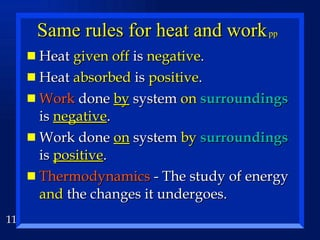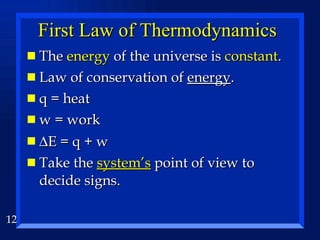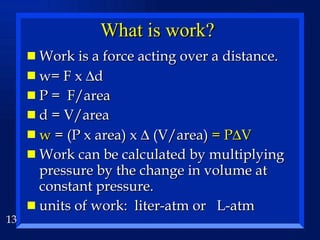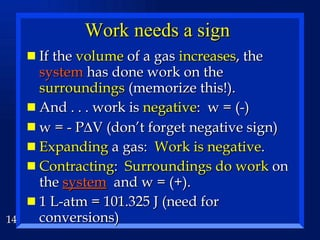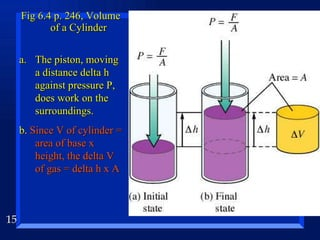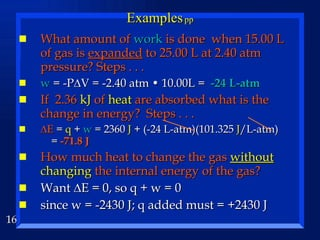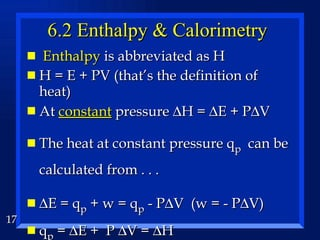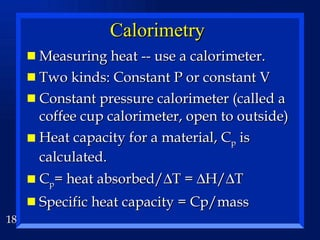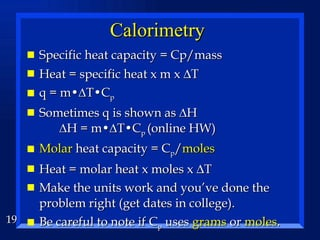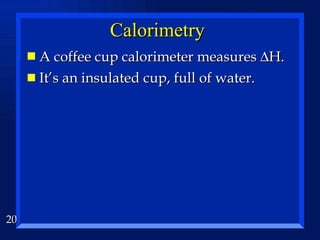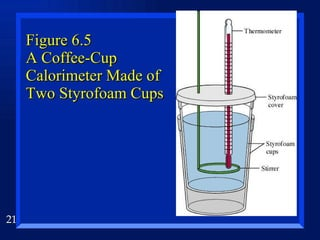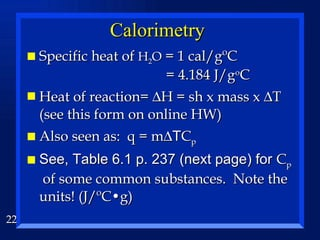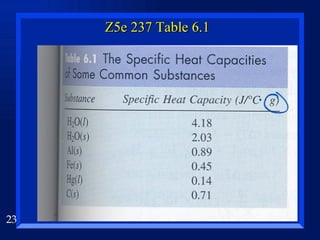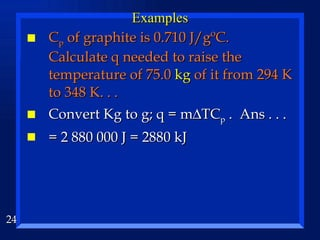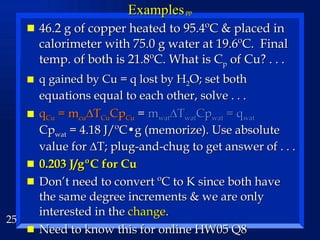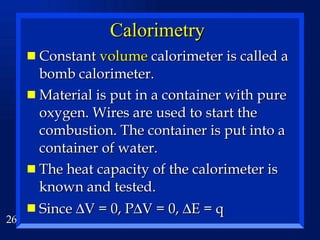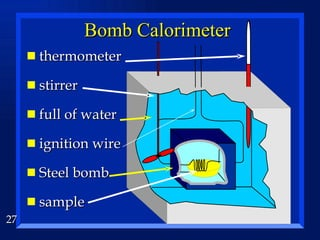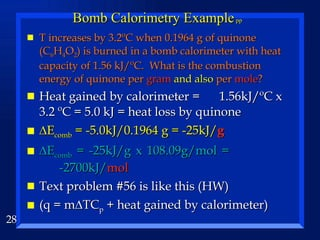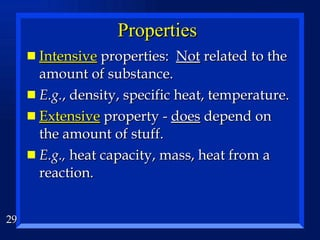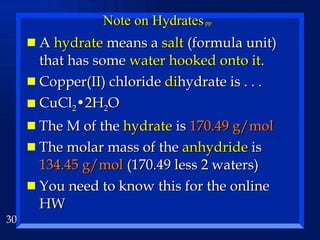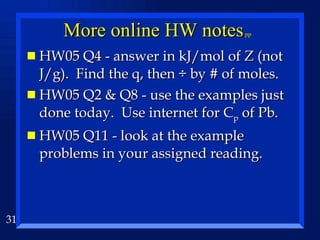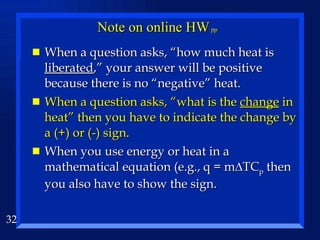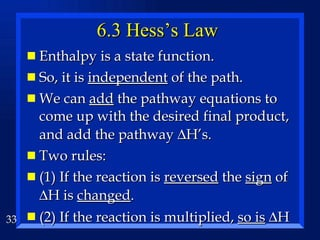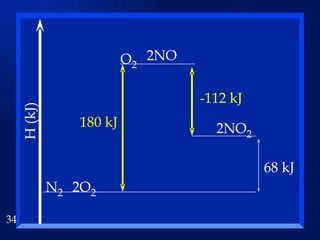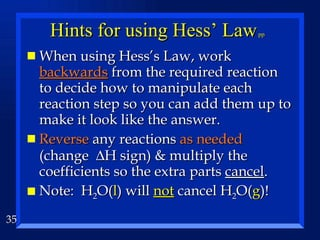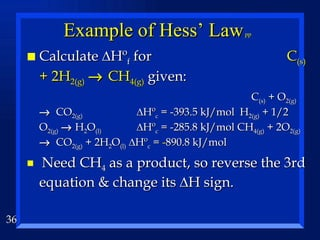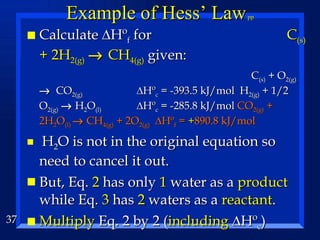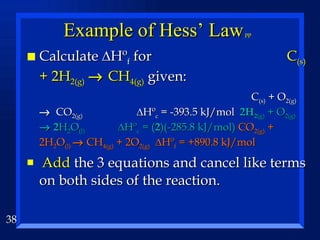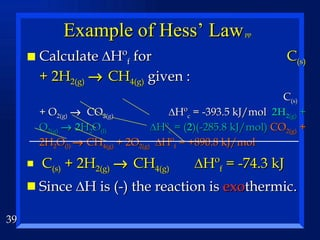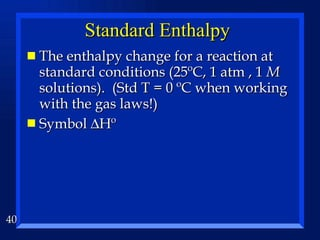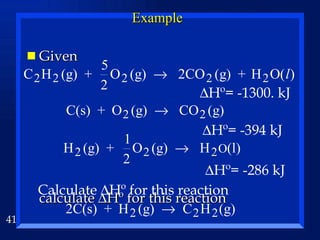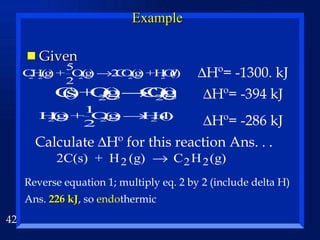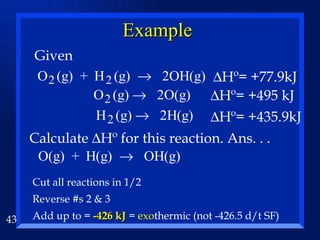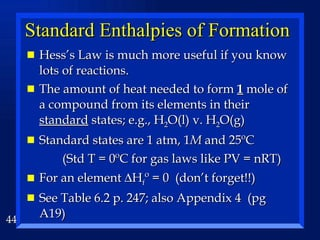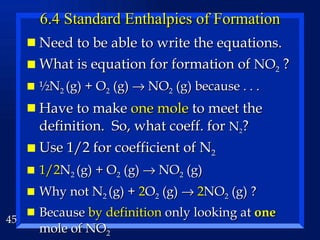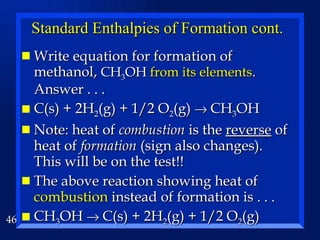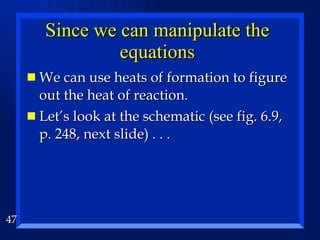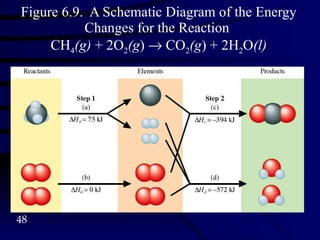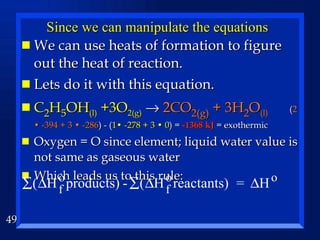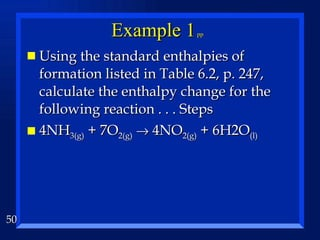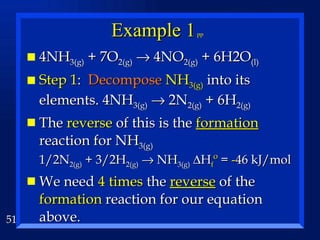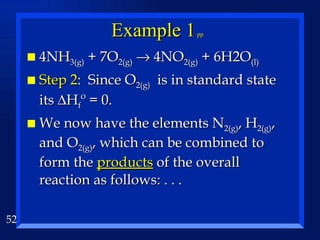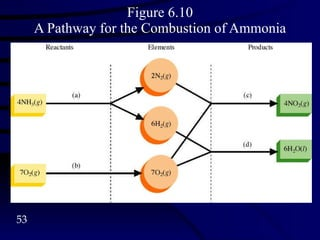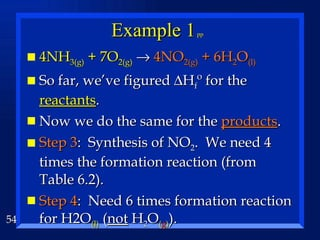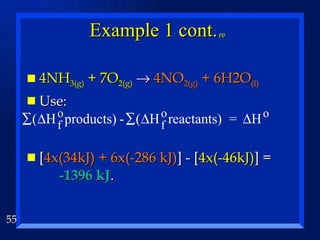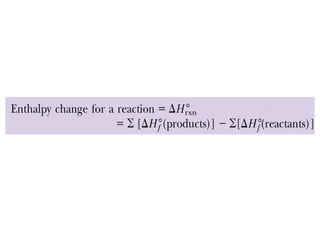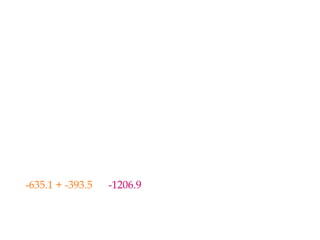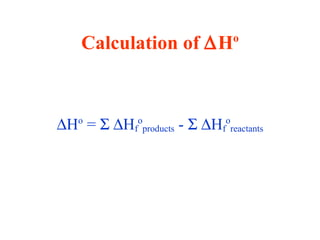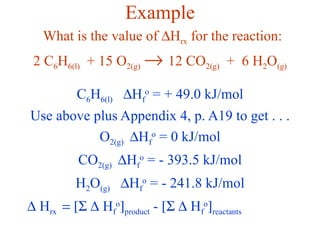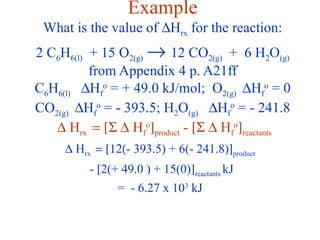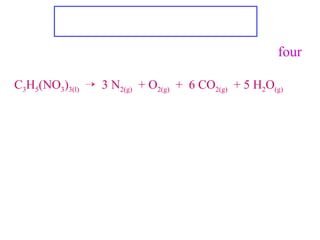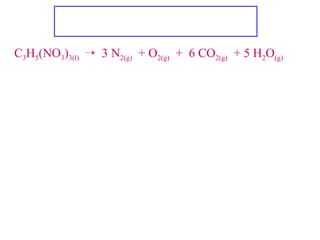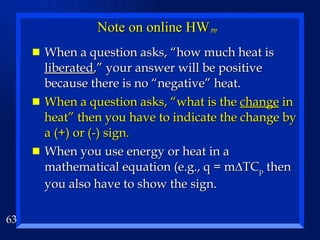1 sur 63

### Ch6 z5e thermo

• 1. Chapter 6 Energy Thermodynamics pp
• 2.
• 3. Enthalpy is a STATE FUNCTION . State functions are PATH-INDEPENDENT
• 4.
• 5.
• 8.
• 11.
• 12.
• 13.
• 14.
• 15.
• 16.
• 17.
• 18.
• 19.
• 20.
• 21. Figure 6.5 A Coffee-Cup Calorimeter Made of Two Styrofoam Cups
• 22.
• 24.
• 25.
• 26.
• 27.
• 28.
• 29.
• 30.
• 31.
• 32.
• 33.
• 34. N 2 2O 2 O 2 2NO 68 kJ 2NO 2 180 kJ -112 kJ H (kJ)
• 35.
• 36.
• 37.
• 38.
• 39.
• 40.
• 41.
• 42.
• 43. Example Given Calculate  Hº for this reaction. Ans. . .  Hº= +77.9kJ  Hº= +495 kJ  Hº= +435.9kJ Cut all reactions in 1/2 Reverse #s 2 & 3 Add up to = -426 kJ = exo thermic (not -426.5 d/t SF)
• 44.
• 45.
• 46.
• 47.
• 48. Figure 6.9. A Schematic Diagram of the Energy Changes for the Reaction CH 4 (g) + 2O 2 (g )  CO 2 (g ) + 2H 2 O (l)
• 49.
• 50.
• 51.
• 52.
• 53. Figure 6.10 A Pathway for the Combustion of Ammonia
• 54.
• 55.
• 56. Calculating  H o reaction using standard enthalpies of formation - Values for  H o f are looked up in tables such as Appendix 4 in your book
• 57. Calculate  H o rxn for CaCO 3(s)  CaO (s) + CO 2(g) compound  H o f (kJ/mol) CaCO 3(s) -1206.9 CaO (s) -635.1 CO 2(g) -393.5 [ -635.1 + -393.5 ] - [ -1206.9 ] = 178.3 = endothermic
• 58.
• 59.
• 60.
• 61. Nitroglycerine is a powerful explosive, giving four different gases when detonated C 3 H 5 (NO 3 ) 3(l)  3 N 2(g) + O 2(g) + 6 CO 2(g) + 5 H 2 O (g) Given that  H o f for nitroglycerine is -364 kJ/mol, and consulting a table for the enthalpies of formation of the other compounds, calculate the enthalpy change when 10.0 g of nitroglycerine is detonated.  H o rxn and Stoichiometry Find enthalpy for 1 mole of NTG (use previous method) Then convert 10.0 g NTG to moles and use mole ratio between that and 1 mole. 10g ÷ 227 g/mol = 0.044 mol
• 62. C 3 H 5 (NO 3 ) 3(l)  3 N 2(g) + O 2(g) + 6 CO 2(g) + 5 H 2 O (g) calculate ∆H rxn when 10.0 g of NTG is detonated.  H o rxn and Stoichiometry [6•-394 + 5•-242] - [-364] = -3.21 x 10 3 kJ/mol This is for 1 mol. Have 10.0 g, convert to moles 10g ÷ 227 g/mol = 0.044 mol Then 0.044 mol • -3.21 x 10 3 kJ = -141 kJ exothermic
• 63.

### Notes de l'éditeur

1. Z5e 242 Section 6.1 - Nature of Energy
2. Z5e 243
3. Z5e Rf. Fig 6.2 p. 244
4. Rf. Fig 6.3 p. 245
5. Z5e 245
6. Z5e 245
7. Z5e 245
8. Z5e 244
9. d = distance
10. Z5e Fig. 6.4 The piston, moving a distance delta h against pressure P, does work on the surroundings. Since V of cylinder = area of base x height, the delta V of gas = delta h x A
11. w = -p(chg V) = -24 l-atm E = q + w = 2360 J + (-24 l-atm)(101.325 j/l-atm) = -71.8 J Want E = 0, so q + w = 0, so since w = -2430 J; q added must = + 2430 J
12. Z5e 248 Section 6.2 Enthalpy &amp; Calorimetry
13. Z5e 250
14. Z5e 251
15. Convert Kg to g; q = m  TC p = 2 880 000 J = 2880 kJ q gained = q lost; set both equations equal to each other. Ans. 0.203 J/g o C Be sure to discuss that we are looking at change in T, so since C and K degree increments are equal, do NOT convert change in T value to Kelvin. E.g., 10 Kelvin degree change should not be converted to a minus 263 Celsius change.
16. Convert Kg to g; q = m  TC p = 2 880 000 J = 2880 kJ q gained = q lost; set both equations equal to each other. Ans. 0.203 J/g o C Be sure to discuss that we are looking at change in T, so since C and K degree increments are equal, do NOT convert change in T value to Kelvin. E.g., 10 Kelvin degree change should not be converted to a minus 263 Celsius change.
17. Z5e 252
18. Z5e 253 and see Fig. 6.6 p. 254
19. Z5e 284 q. 50
20. (2 * -394 + 3 * -286) - (1 *-278 - 3 * 0) = -1368 kJ/mol = exothermic Oxygen = O since element; liquid water value is not same as gaseous water
21. Z5e 256 6.3 Hess’ Law
22. Z5e 257 Fig. 6.7 - same change occurs whether in 1 or 2 steps. N 2 + 2O 2 --&gt; 2NO 2 ;  H 1 = 68kJ Or 2 step: N 2 + O 2 --&gt; 2NO ;  H 2 = 180kJ 2NO + O 2 --&gt; 2NO 2 l;  H 3 = -112kJ N 2 + 2O 2 --&gt; 2NO 2 ;  H 1 = 68kJ
23. Z5e 259
24. Hrw 520
25. Hrw 520
26. Hrw 520
27. Hrw 520
28. Reverse equation 1; multiply eq. 2 by 2 (include delta H) Ans. 226 kJ, so endothermic
29. Reverse equation 1; multiply eq. 2 by 2 (include delta H) Ans. 226 kJ, so endothermic
30. Cut all rxns in 1/2 Reverse #s 2 &amp; 3 Add up to = -426 kJ = exothermic
31. Z5e Section 6.4 Standard Enthalpies of Formation
32. Z53 261 a. Use 1/2 for coefficient of N 2 b. C(s) + 2H 2 (g) + 1/2 O 2 (g) = CH 3 OH
33. Z53 261 a. Use 1/2 for coefficient of N 2 b. C(s) + 2H 2 (g) + 1/2 O 2 (g) = CH 3 OH
34. Z5e 263
35. (2 * -394 + 3 * -286) - (1 *-278 - 3 * 0) = -1368 kJ/mol = exothermic Oxygen = O since element; liquid water value is not same as gaseous water
36. Z5e 264 SE 6.9
37. Z5e 264 SE 6.9
38. Z5e 264 SE 6.9
39. Z5e 264 Fig. 6.10 used with SE 6.9.
40. Z5e 264 SE 6.9
41. (2 * -394 + 3 * -286) - (1 *-278 - 3 * 0) = -1368 kJ/mol = exothermic Oxygen = O since element; liquid water value is not same as gaseous water
42. Hrw 523
43. (2 * -394 + 3 * -286) - (1 *-278 - 3 * 0) = -1368 kJ/mol = exothermic Oxygen = O since element; liquid water value is not same as gaseous water